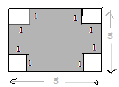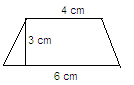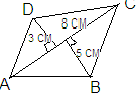Courses

# Test: Mensuration- 1

## 40 Questions MCQ Test Mathematics (Maths) Class 8 | Test: Mensuration- 1

Description
This mock test of Test: Mensuration- 1 for Class 8 helps you for every Class 8 entrance exam. This contains 40 Multiple Choice Questions for Class 8 Test: Mensuration- 1 (mcq) to study with solutions a complete question bank. The solved questions answers in this Test: Mensuration- 1 quiz give you a good mix of easy questions and tough questions. Class 8 students definitely take this Test: Mensuration- 1 exercise for a better result in the exam. You can find other Test: Mensuration- 1 extra questions, long questions & short questions for Class 8 on EduRev as well by searching above.
QUESTION: 1

### In fig., a square of side 5 cm, the area of shaded portion isSolution:

Area of square having sides 5 cm = 52 = 25 cm2

Area of smaller square having sides 1 cm = 12 = 1 cm2

Then area of 4 squares will be 4 cm2

Area of shaded portion will be =  Area of square having sides 5 cm - area of 4  smaller squares (Unshaded portion)

Area of shaded portion = 25 cm- 4 cm2

Area of shaded portion = 21 cm2

QUESTION: 2

Solution:
QUESTION: 3

### The area of a trapezium is

Solution:
QUESTION: 4

In a quadrilateral, half of the product of the sum of the lengths of parallel sides  and the parallel distance between them gives the area of

Solution:
QUESTION: 5

The area of rhombus is

Solution: D1 , D2 stands for the two diagonal in a rhombus, so we 1/2*d1*d2
QUESTION: 6

Which of the following has the formula ½ (sum of parallel sides)×h

Solution:
QUESTION: 7

The length of parallel sides of trapezium is 14 cm and 6 cm and its height is 5 cm. Its area will be

Solution:
QUESTION: 8

The area of trapezium in the adjoining figure isSolution:
QUESTION: 9

Which of the following is an example of two dimensions

Solution:

2d shapes are on the plane . The circle is not in space ,it is in plane. So it is a 2d shape.

QUESTION: 10

Which of the following shape has two dimensions

Solution:

Chalk box and soap have breadth,length and height . Cylinder has 2 radii and height. A ring (here) is made up of two concentric circles which is a 2d figure. Hence ring is 2 dimensional

QUESTION: 11

Two dimensional figure is a

Solution: A two-dimensional figure, also called a plane or planar figure, is a set of line segments or sides and curve segments or arcs, all lying in a single plane. The sides and arcs are called the edges of the figure. The edges are one-dimensional, but they lie in the plane, which is two-dimensional.
QUESTION: 12

Plane figures are

Solution:
QUESTION: 13

Solid figures are

Solution:
QUESTION: 14

Which of the following is an example of 3 D

Solution:

3D figures appear in space. Since square,triangle and rectangle appear on plain, that is, paper, sphere is the only #D object.

QUESTION: 15

Pyramid is an example of

Solution:
QUESTION: 16

In a right circular cylinder, the line segments joining the centre of circular faces is ________  to the base

Solution:
QUESTION: 17

The amount of space occupied by a three dimensional objects is called its

Solution:
QUESTION: 18

The standard unit of volume is

Solution:
QUESTION: 19

The formula for finding the surface area of cube is

Solution:

Total surface area of cube = 6a2

Curved surface area of cube = 4a

Volume of cube = a2

QUESTION: 20

If the side of the cube is 2 m, then the surface area of the cube is

Solution:
QUESTION: 21

The formula for finding total surface area of cuboid is

Solution:
QUESTION: 22

The formula for lateral surface area of cuboid is

Solution:
QUESTION: 23

The area of four walls of the room is

Solution:

Since we have area of wall as b*h and h*l .So area of 4 walls is 2h*b+2h*l=2h(b+l)

QUESTION: 24

The formula for volume of cube is

Solution:
QUESTION: 25

The quantity that a container holds is called its

Solution:
QUESTION: 26

1 m3 is  ______________ .

Solution:
QUESTION: 27

The height of cuboid  whose volume is 200 cm3 and base  area is 20 cm2 is

Solution:
QUESTION: 28

1 m l = ___________ .

Solution:

1m= 1000 L and 1m3=1000000cmand 1000L= 1000000ml
So 1000000cm= 1000000ml which is 1ml = 1cm3

QUESTION: 29

If each edge of a cube is doubled, its surface are will increase

Solution:
QUESTION: 30

The formula for finding total surface area of cylinder is

Solution:

Total surface area of a cylinder = πr2+2πrh+πr2=2πr(r+h)

QUESTION: 31

The formula for finding lateral surface area  of cylinder is

Solution:
QUESTION: 32Solution:
QUESTION: 33

If the diagonals of rhombus are 6 cm & 8 cm, its area will be

Solution:

Area of rhombus is ½* d1*d2 =6*8/2=24cm2.

QUESTION: 34

Diagonals of rhombus are

Solution:
QUESTION: 35

If the base of rhombus of 7 cm and its altitude is 4 cm, its area will be

Solution:
QUESTION: 36

1 L =  __________cm3

Solution:
QUESTION: 37

If the length of edge of cube is 4 cm, its volume is

Solution:
QUESTION: 38

The volume of cuboid of dimensions 4 cm, 2 cm and 3 cm is

Solution:
QUESTION: 39

The formula for finding volume of cuboid is

Solution:
QUESTION: 40

The formula for finding volume of cylinder  is

Solution:
The volume of the cylinder is a measurement describing how much (in cubic units) the cylinder will hold. It is a measure of the space inside the cylinder.
The formula for finding the Volume of a right circular cylinder is:
V = πr^2 h,
where r is the radius of the circle at one base of the cylinder,
and h is the height of the cylinder (the distance between the bases.)